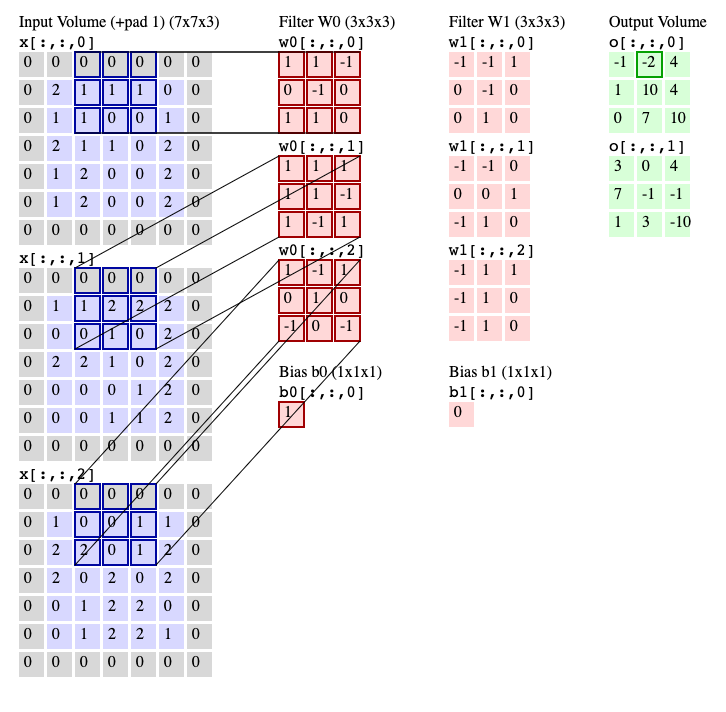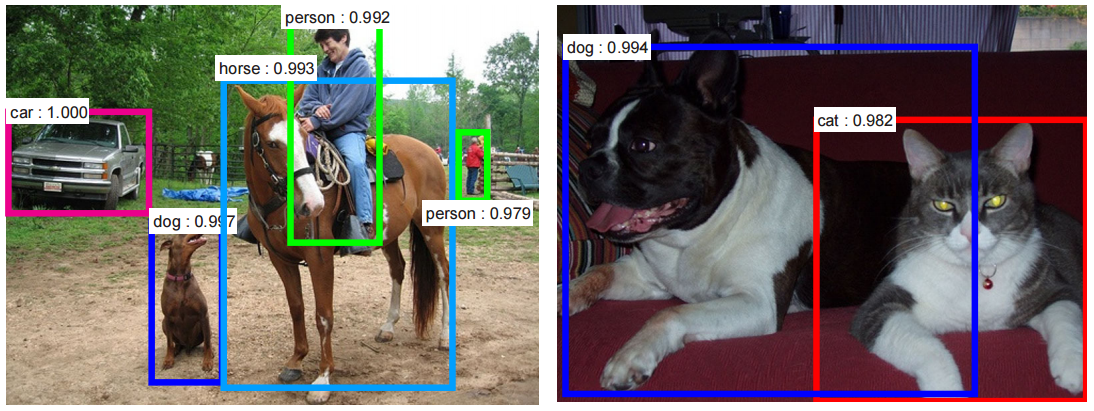# Convolutional Neural Networks

## What is a Convolutional Neural Network?

A convolutional neural network, or CNN, is a subset of deep learning and neural networks most commonly used to analyze visual imagery. Compared to other image classification algorithms, convolutional neural networks use minimal preprocessing, meaning the network learns the filters that typically are hand-engineered in other systems. Because CNNs operate with such independence from human effort, they offer many advantages over alternative algorithms.

The word convolutional stems from the latin convolvere meaning "to roll together." In mathematics, a convolution is the integral measurement of how two functions overlap as they interact. Similar to the way one might mix two functions by multiplying them together, a convolution exemplifies the changes functions have upon each other. CNNs use this principle in decoding imagery. CNNs analyze the visual elements of the image and attempt to map out the occurrences of matching features.

## How does a Convolutional Neural Network function?

CNNs process images as volumes, receiving a color image as a rectangular box where the width and height are measure by the number of pixels associated with each dimension, and the depth is three layers deep for each color (RGB). These layers are called channels. Within each pixel of the image, the intensity of the R, G, or B is expressed by a number. That number is part of three, stacked two-dimensional matrices that make up the image volume and form the initial data that is fed to into the convolutional network. The network then begins to filter the image by grouping squares of pixels together and looking for patterns, performing what is known as a convolution. This process of pattern analysis is the foundation of CNN functions.Image credit to Andrej Karpathy

## Applications of Convolutional Neural Networks

Convolutional neural networks are a useful resource for other applications outside of machine learning and artificial intelligence. As a powerful tool for image and data analysis, CNNs are used in situations ranging from image recognition, to drug discovery.

### Convolutional Neural Networks and Machine Learning

Convolutional neural networks and machine learning go hand-in-hand. The foundation of CNNs is their ability to operate with minimal human-engineering, aligning well with artificial intelligence. Machine learning relies on the functions of CNNs for processing and analysis of images, language, time series data, and more. Machine learning uses CNNs across a multitude of applications, making it an integral part of artificial intelligence.Image credit to Shaoqing Ren, Kaiming He, Ross Girshick, Jian Sun, Faster R-CNN: Towards Real-Time Object Detection with Region Proposal Networks, arXiv:1506.01497.

### CNNs and GPUs

CNNs have been integral in the performance of modern graphics processing units, also known as GPUs. The integration of CNNs with GPUs in 2004 initially led to an increase of performance 20 times faster than any other comparative GPU. Since then, GPU image recognition has achieved near superhuman performance mainly due to the implementation of convolutional neural networks.

### Text Recognition

Because CNNs are adept at decoding visual imagery, they have proven useful in text analysis. The power of CNNs make natural-language processing possible on analog or handwritten documents, where the images are symbols that need transcription. This function of CNNs is known as optical character recognition, or OCR in short.

### Facial Recognition

As image recognition is a common use for CNNs, facial recognition is a frequent application. As recently as 2015, CNNs have demonstrated the ability to recognize faces from a wide variety of angles, even with limited visibility. The best alternative algorithms struggle with recognizing figures in an image that may be small and thin, but CNNs can handle the task.

### Drug Discovery

CNNs have been applied toward drug discovery. The neural networks process data to understand the interaction between molecules and biological proteins. Much like how image processing CNNs analyze the characteristics of imagery, CNNs for drug discovery train to understand chemical structures. The information that these CNNs provide lead to better potential medical treatments. Notably, the CNN AtomNet was used in the development of treatments for the Ebola virus